# ComputeFest 2019¶

## Convolutional Autoencoders for Image Manipulation¶

Pavlos Protopapas, Vincent Casser, Camilo Fosco $\bullet$ Harvard University

If you have trouble using Google Colab, contact a workshop member and we will use the following link for setting up: https://canvas.harvard.edu/courses/53717

## [Important: Remember to switch runtime to GPU first.]¶

In [ ]:
!git clone https://github.com/Harvard-IACS/2019-computefest.git

In [ ]:
import os
os.chdir("2019-computefest/Wednesday/auto_encoder")

In [ ]:
!ls


## 1. Imports¶

We will import both Keras and the Tensorflow backend. In addition, some common libraries are used for I/O, image and data processing, and visualization.

In :
import keras
from keras import Model
from keras.layers import Input, Conv2D, MaxPooling2D, UpSampling2D, Concatenate, Reshape, Dense, Lambda, Flatten
from keras import backend as K

import warnings
warnings.filterwarnings('ignore')
import numpy as np
import glob
import skimage
import skimage.transform
import skimage.io
import PIL
import numpy as np
import os
from IPython.display import clear_output
import pandas as pd
import matplotlib.pyplot as plt
%matplotlib inline
from ipywidgets import interact, interactive, fixed, interact_manual
import ipywidgets as widgets
import imageio
import utils


## 2. Definitions¶

The major definition here will be our network architecture for the VAE. It follows an encoder-decoder architecture.

In :
def define_encoder_block(x, num_filters):
"""
Todo: Define two sequential 2D convolutional layers (Conv2D) with the following properties:
- num_filters many filters
- kernel_size 3
- activation "relu"
- kernel_initializer "he_normal"
Also define a 2D max pooling layer (MaxPooling2D) (you can keep default arguments).
"""
x = Conv2D(num_filters, 3, activation='relu', padding='same', kernel_initializer='he_normal')(x)
x = Conv2D(num_filters, 3, activation='relu', padding='same', kernel_initializer='he_normal')(x)
x = MaxPooling2D()(x)
return x

In :
def define_decoder_block(x, num_filters):
"""
Todo: Define one 2D upsampling layer (UpSampling2D) (you can keep default arguments).
Also, define two sequential 2D convolutional layers (Conv2D) with the following properties:
- num_filters many filters
- kernel_size 3
- activation "relu"
- kernel_initializer "he_normal"
"""
x = UpSampling2D()(x)
x = Conv2D(num_filters, 3, activation='relu', padding = 'same', kernel_initializer = 'he_normal')(x)
x = Conv2D(num_filters, 3, activation='relu', padding = 'same', kernel_initializer = 'he_normal')(x)
return x

In :
def define_net(variational, height, width, batch_size, latent_dim, conditioning_dim=0,
start_filters=8):
"""Defines a (variational) encoder-decoder architecture.

Args:
variational: Whether a variational autoencoder should be defined.
height: The height of the image input and output.
width: The width of the image input and output.
batch_size: The batchsize that is used during training. Must also be used for inference on the encoder side.
latent_dim: The dimension of the latent space.
conditioning_dim: The dimension of the space of variables to condition on. Can be zero for an unconditional VAE.
start_filters: The number of filters to start from. Multiples of this value are used across the network. Can be used
to change model capacity.

Returns:
Tuple of keras models for full VAE, encoder part and decoder part only.
"""

# Prepare the inputs.
inputs = Input((height, width, 3))
if conditioning_dim > 0:
# Define conditional VAE. Note that this is usually not the preferred way
# of incorporating the conditioning information in the encoder.
condition = Input([conditioning_dim])
condition_up = Dense(height * width)(condition)
condition_up = Reshape([height, width, 1])(condition_up)
inputs_new = Concatenate(axis=3)([inputs, condition_up])
else:
inputs_new = inputs

# Define the encoder.
eblock1 = define_encoder_block(inputs_new, start_filters)
eblock2 = define_encoder_block(eblock1, start_filters*2)
eblock3 = define_encoder_block(eblock2, start_filters*4)
eblock4 = define_encoder_block(eblock3, start_filters*8)
_, *shape_spatial = eblock4.get_shape().as_list()
eblock4_flat = Flatten()(eblock4)

if not variational:
z = Dense(latent_dim)(eblock4_flat)
else:
# Perform the sampling.
def sampling(args):
"""Samples latent variable from a normal distribution using the given parameters."""
z_mean, z_log_sigma = args
epsilon = K.random_normal(shape=(batch_size, latent_dim), mean=0., stddev=1.)
return z_mean + K.exp(z_log_sigma) * epsilon

z_mean = Dense(latent_dim)(eblock4_flat)
z_log_sigma = Dense(latent_dim)(eblock4_flat)
z = Lambda(sampling, output_shape=(latent_dim,))([z_mean, z_log_sigma])

if conditioning_dim > 0:
z_ext = Concatenate()([z, condition])

# Define the decoder.
inputs_embedding = Input([latent_dim + conditioning_dim])
embedding = Dense(np.prod(shape_spatial), activation='relu')(inputs_embedding)
embedding = Reshape(eblock4.shape.as_list()[1:])(embedding)

dblock1 = define_decoder_block(embedding, start_filters*8)
dblock2 = define_decoder_block(dblock1, start_filters*4)
dblock3 = define_decoder_block(dblock2, start_filters*2)
dblock4 = define_decoder_block(dblock3, start_filters)
output = Conv2D(3, 1, activation = 'tanh')(dblock4)

# Define the models.
decoder = Model(input = inputs_embedding, output = output)
if conditioning_dim > 0:
encoder_with_sampling = Model(input = [inputs, condition], output = z)
encoder_with_sampling_ext = Model(input = [inputs, condition], output = z_ext)
vae_out = decoder(encoder_with_sampling_ext([inputs, condition]))
vae = Model(input = [inputs, condition], output = vae_out)
else:
encoder_with_sampling = Model(input = inputs, output = z)
vae_out = decoder(encoder_with_sampling(inputs))
vae = Model(input = inputs, output = vae_out)

# Define the VAE loss.
def vae_loss(x, x_decoded_mean):
"""Defines the VAE loss functions as a combination of MSE and KL-divergence loss."""
mse_loss = K.mean(keras.losses.mse(x, x_decoded_mean), axis=(1,2)) * height * width
kl_loss = - 0.5 * K.mean(1 + z_log_sigma - K.square(z_mean) - K.exp(z_log_sigma), axis=-1)
return mse_loss + kl_loss

if variational:
else:

print('done,', vae.count_params(), 'parameters.')
return vae, encoder_with_sampling, decoder


Next, we define some functions to make encoding and decoding easier.

In :
def encode_image(img, conditioning, encoder, height, width, batch_size):
'''Encodes an image that is given in RGB-channel order with value range of [0, 255].

Args:
img: The image input. If shapes differ from (height, width), it will be resized.
conditoning: The set of values to condition on, if any. Can be None.
encoder: The keras encoder model to use.
height: The target image height.
width: The target image width.
batch_size: The batchsize that the encoder expects.

Returns:
The latent representation of the input image.
'''
if img.shape != height or img.shape != width:
img = skimage.transform.resize(img, (height, width))
img_single = np.expand_dims(img, axis=0)
img_single = img_single.astype(np.float32)
img_single = np.repeat(img_single, batch_size, axis=0)
if conditioning is None:
z = encoder.predict(img_single)
else:
z = encoder.predict([img_single, np.repeat(np.expand_dims(conditioning, axis=0), batch_size, axis=0)])
return z

In :
def decode_embedding(z, conditioning, decoder):
'''Decodes the given representation into an image.

Args:
z: The latent representation.
conditioning: The set of values to condition on, if any. Can be None.
decoder: The keras decoder model to use.
'''
if z.ndim < 2:
z = np.expand_dims(z, axis=0) # Single-batch
if conditioning is not None:
z = np.concatenate((z, np.repeat(np.expand_dims(conditioning, axis=0), z.shape, axis=0)), axis=1)
return decoder.predict(z)

In :
def load_weights(folder):

def save_weights(folder):
if not os.path.isdir(folder):
os.mkdir(folder)
vae.save_weights(folder + '/vae.w')
encoder.save_weights(folder + '/encoder.w')
decoder.save_weights(folder + '/decoder.w')


## Prepare MNIST data¶

Next, we prepare the MNIST data by downsampling it and scaling the intensity ranges. Note that we could have also used binary crossentropy loss here, as MNIST is often used in binarized form. However, we treat the intensity values as floats.

In :
x_train, y_train, x_test, y_test = utils.load_mnist(target_height=32, target_width=32)
# We work on a subset here, to make training on CPU's feasible.
indices_train = np.random.choice(list(range(x_train.shape)), size=30000, replace=False)
x_train = x_train[indices_train]
y_train = y_train[indices_train]
indices_test = np.random.choice(list(range(x_test.shape)), size=2000, replace=False)
x_test = x_test[indices_test]
y_test = y_test[indices_test]

details: shape (60000, 32, 32, 3) min 0.0 max 1.0
details: shape (10000, 32, 32, 3) min 0.0 max 1.0


## 3. Unconditional AE: Application to MNIST¶

First, we define the autoencoder using the definitions above, then we train it for a single epoch:

In :
vae, encoder, decoder = define_net(
False, 32, 32, batch_size=8, latent_dim=2, start_filters=8)

done, 185173 parameters.

In [ ]:
vae.fit(x_train, x_train, batch_size=8, verbose=1, epochs=1)


Alternatively, we can load pre-trained weights.

In :
# load_weights(folder='models/mnist_ae')

In :
# save_weights(folder='models/mnist_ae')


Below, we plot the latent representation of all the MNIST test images and their groundtruth label. As we can see, the clusters are already grouped together quite nicely.

In :
x_test_encoded = encoder.predict(x_test, batch_size=8)
plt.figure(figsize=(6, 6))
plt.scatter(x_test_encoded[:, 0], x_test_encoded[:, 1], c=y_test, cmap='tab10')
plt.colorbar()
plt.show()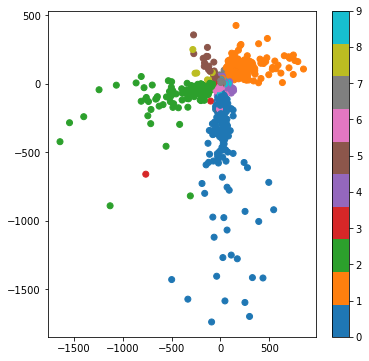In :
x_demo = x_test[np.random.randint(x_test.shape)]
pred = vae.predict(np.expand_dims(x_demo, axis=0))
plt.imshow(x_demo)
plt.show()
plt.imshow(pred)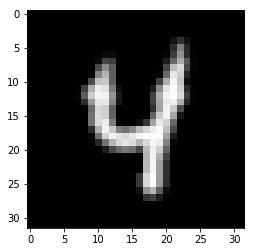Out:
<matplotlib.image.AxesImage at 0x202f2ac80f0>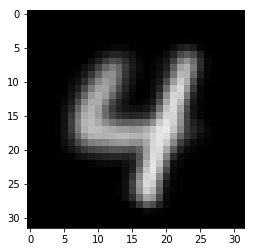## 4. Unconditional VAE: Application to MNIST¶

First, we define the VAE network using the definitions above, then we train it for a single epoch:

In :
vae, encoder, decoder = define_net(
True, 32, 32, batch_size=8, latent_dim=2, start_filters=4)

done, 47055 parameters.

In [ ]:
vae.fit(x_train, x_train, batch_size=8, verbose=1, epochs=1)


Alternatively, we can load pre-trained weights.

In :
# load_weights(folder='models/mnist_vae')

In :
# save_weights(folder='models/mnist_vae')


After just one epoch, we already get promising results. Below, we plot the latent representation of all the MNIST test images and their groundtruth label. As we can see, the clusters are already grouped together quite nicely.

In :
x_test_encoded = encoder.predict(x_test, batch_size=8)
plt.figure(figsize=(6, 6))
plt.scatter(x_test_encoded[:, 0], x_test_encoded[:, 1], c=y_test, cmap='tab10')
plt.colorbar()
plt.show()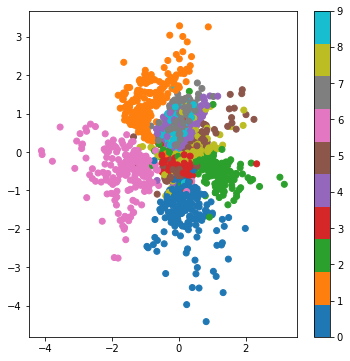We also render parts of the manifold by varying the latent representation.

In :
_ = utils.display_manifold(decoder,
32,
32,
bound_x=5,
bound_y=5,
base_vec=np.array([0,0]),
desc_x='latent_dim1',
desc_y='latent_dim2',
file_out='rendering_mnist.png')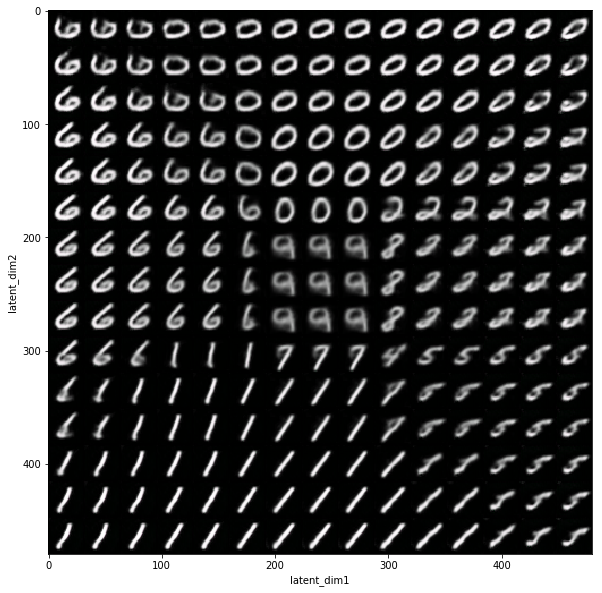## 5. Conditional VAE: Application to CelebA¶

For the face dataset CelebA, we will use a conditional VAE. We first define the hyperparameters to use for this experiment.

In :
VARIATIONAL = True
HEIGHT = 128
WIDTH = 128
BATCH_SIZE = 16
LATENT_DIM = 16
START_FILTERS = 32
CONDITIONING = True


Since this is a large dataset, we also define a custom data generator to avoid caching the whole set in memory.

In :
class CustomDataGenerator(keras.utils.Sequence):
def __init__(self, files, batch_size, target_height, target_width, conditioning_dim=0, conditioning_data=None):
'''
Intializes the custom generator.

Args:
files: The list of paths to images that should be fed to the network.
batch_size: The batchsize to use.
target_height: The target image height. If different, the images will be resized.
target_width: The target image width. If different, the images will be resized.
conditioning_dim: The dimension of the conditional variable space. Can be 0.
conditioning_data: Optional dictionary that maps from the filename to the data to be
conditioned on. Data must be numeric. Can be None. Otherwise, len must be equal to
conditioning_dim.
'''
self.files = files
self.batch_size = batch_size
self.target_height = target_height
self.target_width = target_width
self.conditioning_dim = conditioning_dim
self.conditioning_data = conditioning_data

def on_epoch_end(self):
'''Shuffle list of files after each epoch.'''
np.random.shuffle(self.files)

def __getitem__(self, index):
cur_files = self.files[index*self.batch_size:(index+1)*self.batch_size]
# Generate data
X, y = self.__data_generation(cur_files)
return X, y

def __data_generation(self, cur_files):
X = np.empty(shape=(self.batch_size, self.target_height, self.target_width, 3))
Y = np.empty(shape=(self.batch_size, self.target_height, self.target_width, 3))
if self.conditioning_data != None:
C = np.empty(shape=(self.batch_size, self.conditioning_dim))

for i, file in enumerate(cur_files):
if img.shape != self.target_height or img.shape != self.target_width:
img = skimage.transform.resize(img, (self.target_height, self.target_width)) # Resize.
img = img.astype(np.float32) / 255.
X[i] = img
Y[i] = img
if self.conditioning_data != None:
C[i] = self.conditioning_data[os.path.basename(file)]

if self.conditioning_data != None:
return [X, C], Y
else:
return X, Y

def __len__(self):
return int(np.floor(len(self.files) / self.batch_size))


We will read the annotation data now.

In :
# Find image files.
files = glob.glob('celeba/img_align_celeba/*.jpg')
print(len(files), 'images found.')

columns = df.columns

202599 images found.

Out:
Filename 5_o_Clock_Shadow Arched_Eyebrows Attractive Bags_Under_Eyes Bald Bangs Big_Lips Big_Nose Black_Hair ... Sideburns Smiling Straight_Hair Wavy_Hair Wearing_Earrings Wearing_Hat Wearing_Lipstick Wearing_Necklace Wearing_Necktie Young
0 000001.jpg -1 1 1 -1 -1 -1 -1 -1 -1 ... -1 1 1 -1 1 -1 1 -1 -1 1
1 000002.jpg -1 -1 -1 1 -1 -1 -1 1 -1 ... -1 1 -1 -1 -1 -1 -1 -1 -1 1
2 000003.jpg -1 -1 -1 -1 -1 -1 1 -1 -1 ... -1 -1 -1 1 -1 -1 -1 -1 -1 1

3 rows × 41 columns

We convert the dataframe into a dictionary, mapping from filename to the individual image attributes. We then instantiate the generator.

In :
dd = {}
selected_conditionals = list(columns[1:])
for i, row in df.iterrows():
dd[row['Filename']] = [int(row[c]) for c in selected_conditionals]

gen = CustomDataGenerator(files=files,
batch_size=BATCH_SIZE,
target_height=HEIGHT,
target_width=WIDTH,
conditioning_dim=len(selected_conditionals),
conditioning_data=dd if CONDITIONING else None)


Finally, we define the conditional VAE and start the training.

In :
vae, encoder, decoder = define_net(variational=VARIATIONAL,
height=HEIGHT,
width=WIDTH,
batch_size=BATCH_SIZE,
latent_dim=LATENT_DIM,
conditioning_dim=len(selected_conditionals) if CONDITIONING else 0,
start_filters=START_FILTERS)

done, 5063811 parameters.

In [ ]:
# vae.fit_generator(gen, verbose=1, epochs=20)


Alternatively, we can load pre-trained weights:

In :
load_weights(folder='models/celeba_vae')

In :
# save_weights(folder='models/celeba_vae')


Let's look at some examples. First, we will select a random image from the CelebA dataset, and read the related annotation data.

In :
rnd_file = np.random.choice(files)
file_id = os.path.basename(rnd_file)
init_meta = dd[file_id]
plt.imshow(img)
plt.show()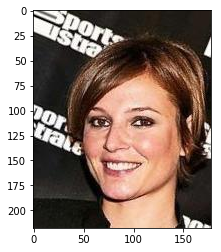Now, we will encode the image into its latent representation.

In :
z = encode_image(img.astype(np.float32) / 255., np.array(init_meta), encoder, HEIGHT, WIDTH, BATCH_SIZE)
print('latent sample:\n', z)

latent sample:
[-1.3673916   1.2208009   0.06379721 -1.0093268   1.1415257   2.6127234
-1.9030862  -3.1780279  -0.7717539  -0.16299067 -0.50487703  1.0116105
0.1154899   1.1115322   0.6290148   0.23716433]


Decoding the latent representation should result in a face with somewhat similar characterics.

In :
ret = decode_embedding(z, init_meta, decoder)
plt.imshow(ret)
plt.show()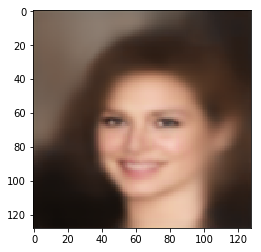The next step is interesting: We can manipulate the embedding to change the facial structure. We can modify both our conditionals, as well as the latent variables. Here, we plot sliders to make the process interactive.

In :
# Note: Interactive widgets (i.e. this cell) will not work in Colab.

def render(**params):
perturb_latent = np.zeros(shape=(LATENT_DIM))
for i in range(LATENT_DIM):
perturb_latent[i] = params['latent' + str(i)]

c = []
for k,v in params.items():
if not k.startswith('latent'):
c.append(v)
ret = decode_embedding(z + perturb_latent, conditioning=np.array(c), decoder=decoder)
ret = np.clip(ret, 0, 1)
plt.figure(figsize=(3,3))
plt.imshow(ret)

lower, upper = -10, 10
params = {}
for i, c in enumerate(selected_conditionals):
params[c] = widgets.FloatSlider(min=lower,max=upper,step=0.1,value=init_meta[i], layout=widgets.Layout(width='70%', height='20px'))
for c in ['latent' + str(i) for i in range(LATENT_DIM)]:
params[c] = widgets.FloatSlider(min=lower,max=upper,step=0.1,value=0,layout=widgets.Layout(width='70%', height='20px'))

interactive_plot = interactive(render, **params)
output = interactive_plot.children[-1]
output.layout.height = '200px'
interactive_plot


### Play around with the different values and see what it generates!¶

In :
# Modify the following variables to change the face generated below.
# Set them to numbers between roughly -10 and 10.
# Large values might seem unrealistic, so try with small numbers first.
# If you set them to None, they will take the default values.

smiling = None # e.g. change this to -3 or 3 and see the results!
male = None
no_beard = None
attractive = None
bald = None
chubby = None
eyeglasses = None
young = None

# ------------------------------------
# Don't change the code below.
# It introduces the values you defined in the conditional vector
# that is used by the VAE.

meta = init_meta.copy()
meta = attractive if attractive else init_meta
meta = bald if bald else init_meta
meta = chubby if chubby else init_meta
meta = eyeglasses if eyeglasses else init_meta
meta = male if male else init_meta
meta = no_beard if no_beard else init_meta
meta = smiling if smiling else init_meta
meta = young if young else init_meta

ret = decode_embedding(z, meta, decoder)
plt.imshow(ret)
plt.show()

print('Attractive:', meta)
print('Bald:', meta)
print('Chubby:', meta)
print('Eyeglasses:', meta)
print('Male:', meta)
print('No_Beard:', meta)
print('Smiling:', meta)
print('Young:', meta)